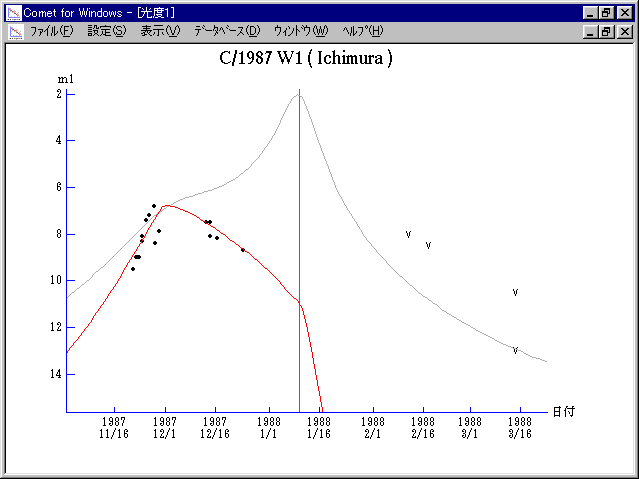# C/1987 W1 ( Ichimura )###Profile

 Discovery Date November 22, 1987 Magnitude 9 mag Discoverer Yoshimi Ichimura (Yoshimi-machi, Saitama)

###Orbital Elements

```          T = 1988 Jan. 10.100 ET    W = 329.317
O = 225.800   1950.0
q =   0.19951 AU           i =  41.564
```

###Magnitudes Graph

```        m1 =  8.0 + 5 log d + 25   log r  [   ,-40]  (             - 1987 Dec.  1)
m1 =  9.5 + 5 log d -  2.0 log r  [-40,  0]  (1987 Dec.  1 - 1988 Jan. 10)
m1 = 25   + 5 log d + 20   log r  [  0,   ]  (1988 Jan. 10 -             )

* Gray curve is:  m1 = 9.0 + 5 log d + 10.0 log r
```##### The orbital elements are printed on IAUC 4504. The magnitudes graphs are made with Comet for Windows.